# Depreciation

Table of Contents What Is Depreciation? If a company buys a piece of equipment for $50,000, it may expense its entire cost in year one or write the value of the asset off over the asset's 10-year useful life. Using these variables, the accountant calculates depreciation expense as the difference between the cost of the asset and its salvage value, divided by the useful life of the asset. It reports equal depreciation expense each year throughout the entire useful life until the entire asset is depreciated to its salvage value. The basic difference between depreciation expense and accumulated depreciation lies in the fact that one appears as an expense on the income statement while the other is a contra asset reported on the balance sheet.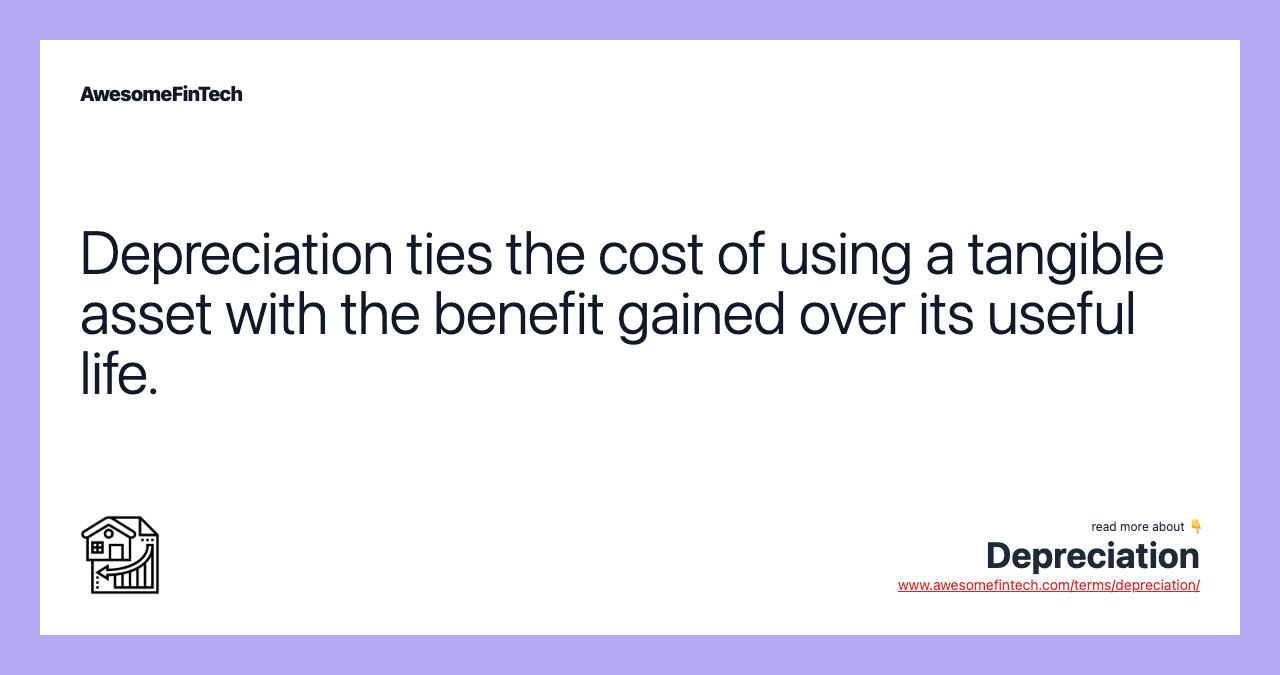## More in Business ## What Is Depreciation? The term depreciation refers to an accounting method used to allocate the cost of a tangible or physical asset over its useful life or life expectancy. Depreciation represents how much of an asset's value has been used. Depreciating assets helps companies earn revenue from an asset while expensing a portion of its cost each year the asset is in use. Not accounting for depreciation can greatly affect a company's profits. Companies can also depreciate long-term assets for both tax and accounting purposes.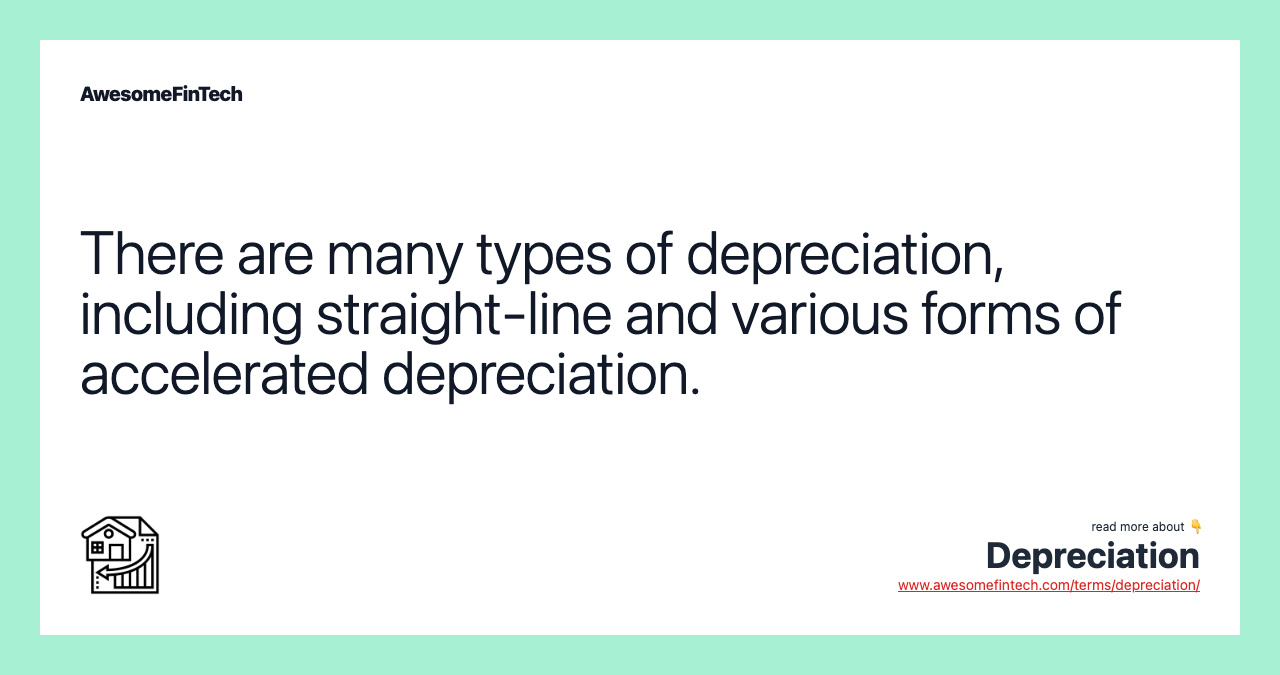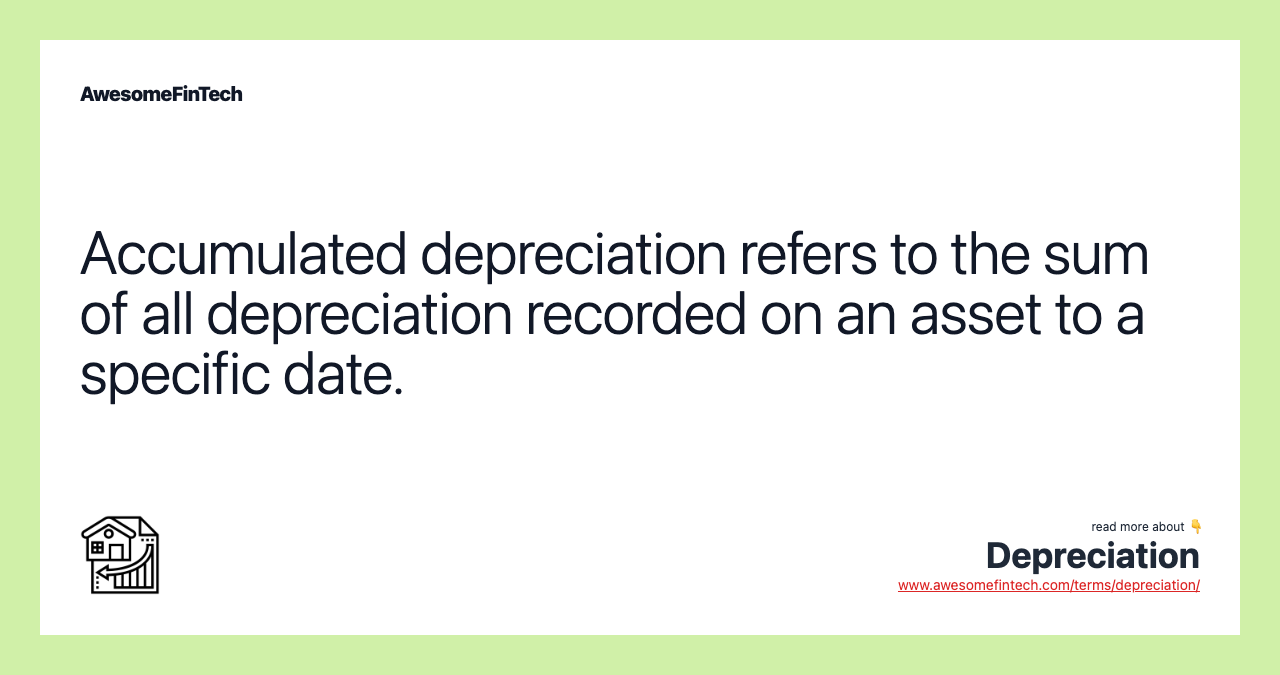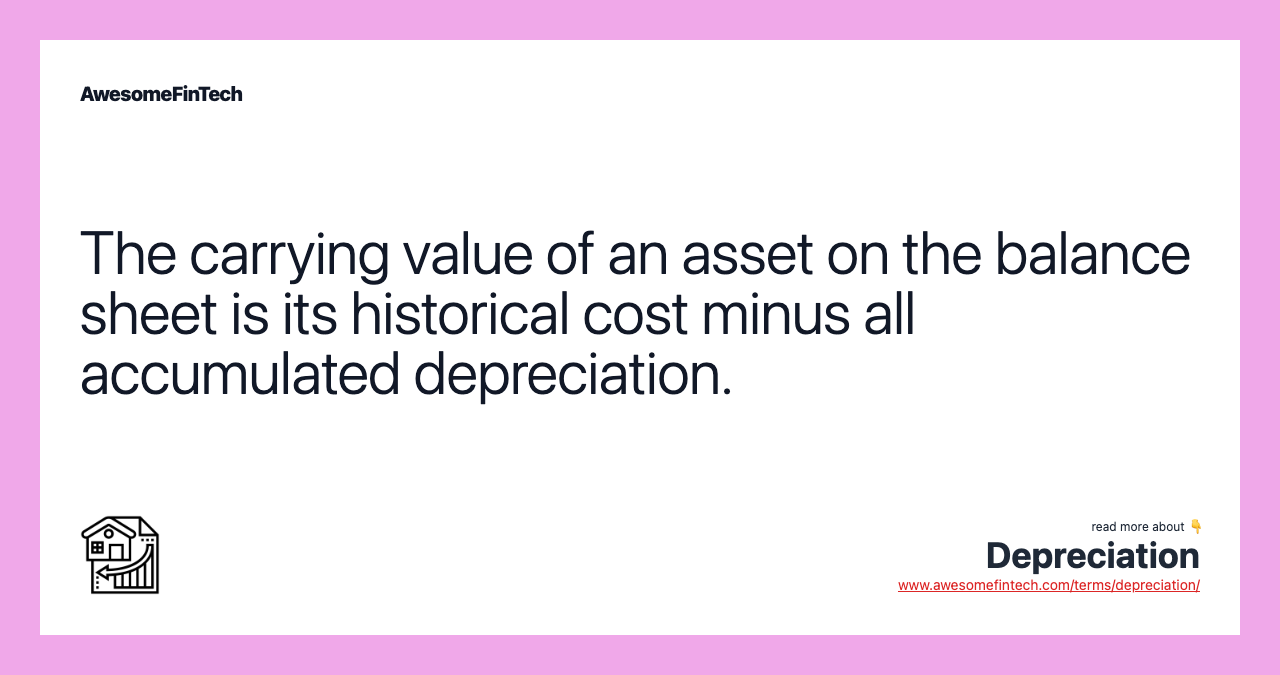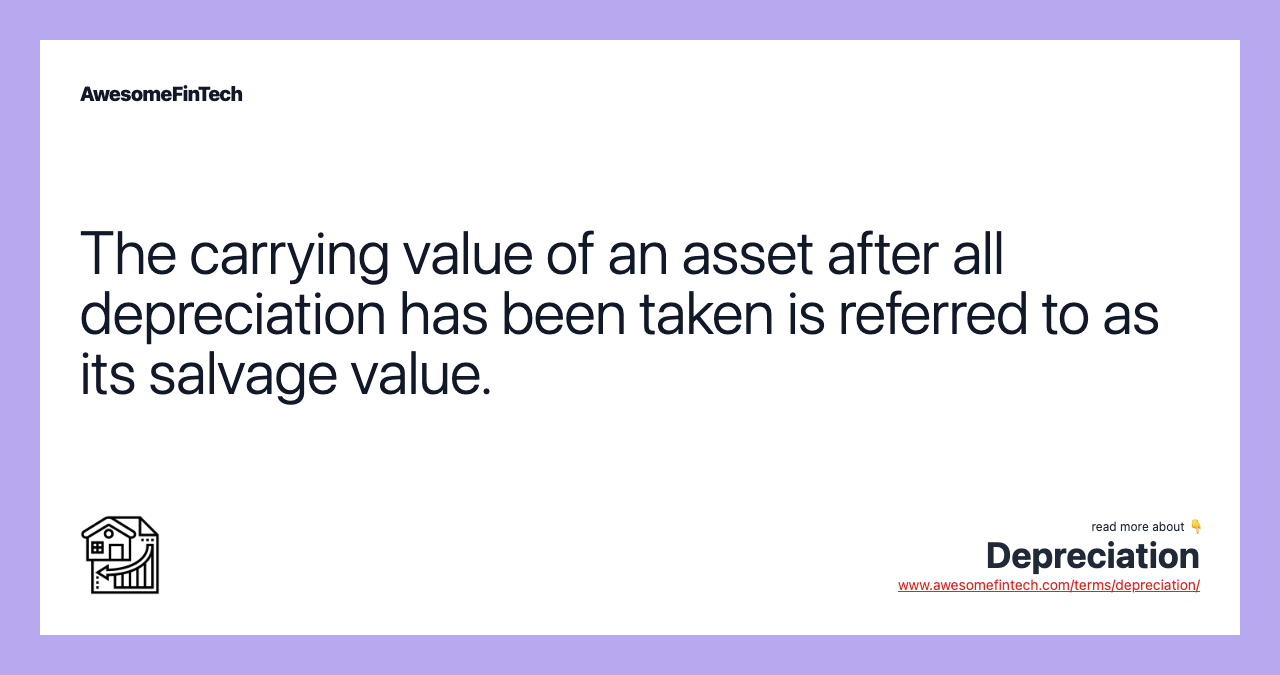## Understanding Depreciation Assets such as machinery and equipment are expensive. Instead of realizing an asset's entire cost in year one, companies can use depreciation to spread out the cost and generate revenue from it. This is done through depreciation, which allows a company to write off an asset's value over a period of time, notably its useful life. It may be used to account for declines over time in the carrying value, which represents the difference between the original cost and the accumulated depreciation of the years. Depreciation is taken regularly so a company can move the asset's cost from the balance sheet to the income statement. When a company buys an asset, it records the transaction as a debit to increase an asset account on the balance sheet and a credit to reduce cash (or increase accounts payable), which is also on the balance sheet. Neither journal entry affects the income statement, where revenues and expenses are reported. At the end of an accounting period, an accountant books depreciation for all capitalized assets that are not fully depreciated. The journal entry consists of a: As noted above, businesses can take advantage of depreciation for both tax and accounting purposes. This means they can take a tax deduction for the cost of the asset, reducing taxable income. But the Internal Revenue Service (IRS) states that when depreciating assets, companies must spread the cost out over time. The IRS also has rules for when companies can take a deduction. The IRS publishes depreciation schedules detailing the number of years an asset can be depreciated for tax purposes, based on various asset classes. ## Special Considerations Depreciation is considered a non-cash charge since it doesn't represent an actual cash outflow. The entire cash outlay might be paid initially when an asset is purchased, but the expense is recorded incrementally for financial reporting purposes. That's because assets provide a benefit to the company over a lengthy period of time. But the depreciation charges still reduce a company's earnings, which is helpful for tax purposes. The matching principle under generally accepted accounting principles (GAAP) is an accrual accounting concept that dictates that expenses must be matched to the same period in which the related revenue is generated. Depreciation helps to tie the cost of an asset with the benefit of its use over time. In other words, the asset is put to use each year and generates revenue — the incremental expense associated with using up the asset is also recorded. The total amount depreciated each year, which is represented as a percentage, is called the depreciation rate. For example, if a company had$100,000 in total depreciation over the asset's expected life, and the annual depreciation was $15,000. This means the rate would be 15% per year. The depreciation rate is used in both the declining balance and double-declining balance calculations. ### Threshold Amounts Different companies may set their own threshold amounts for when to begin depreciating a fixed asset or property, plant, and equipment (PP&E). For example, a small company may set a$500 threshold, over which it depreciates an asset. On the other hand, a larger company may set a $10,000 threshold, under which all purchases are expensed immediately. ### Accumulated Depreciation Accumulated depreciation is a contra asset account, meaning its natural balance is a credit that reduces the net asset value (NAV). Accumulated depreciation on any given asset is its cumulative depreciation up to a single point in its life. As stated earlier, carrying value is the net of the asset account and the accumulated depreciation. The salvage value is the carrying value that remains on the balance sheet after which all depreciation is accounted for until the asset is disposed of or sold. It is based on what a company expects to receive in exchange for the asset at the end of its useful life. An asset’s estimated salvage value is an important component in the calculation of depreciation. ## Types of Depreciation ### Straight-Line Depreciating assets using the straight-line method is the most basic way to record depreciation. It reports equal depreciation expense each year throughout the entire useful life until the entire asset is depreciated to its salvage value. Let's assume that a company buys a machine at a cost of$5,000. The company decides on a salvage value of $1,000 and a useful life of five years. Based on these assumptions, the depreciable amount is$4,000 ($5,000 cost -$1,000 salvage value).

The annual depreciation using the straight-line method is calculated by dividing the depreciable amount by the total number of years. In this case, it amounts to $800 per year ($4,000 ÷ 5). This results in a depreciation rate of 20% ($800÷$4,000).

### Declining Balance

The declining balance method is an accelerated depreciation method. This method depreciates the machine at its straight-line depreciation percentage times its remaining depreciable amount each year. Because an asset's carrying value is higher in earlier years, the same percentage causes a larger depreciation expense amount in earlier years, declining each year.

Using the straight-line example above, the machine costs $5,000, has a salvage value of$1,000, a five-year life, and is depreciated at 20% each year, so the expense is $800 in the first year ($4,000 depreciable amount x 20%), $640 in the second year (($4,000 - $800) x 20%), and so on. ### Double Declining Balance (DDB) The double-declining balance (DDB) method is another accelerated depreciation method. After taking the reciprocal of the useful life of the asset and doubling it, this rate is applied to the depreciable base, book value, for the remainder of the asset’s expected life. For example, an asset with a useful life of five years would have a reciprocal value of 1/5 or 20%. Double the rate, or 40%, is applied to the asset's current book value for depreciation. Although the rate remains constant, the dollar value will decrease over time because the rate is multiplied by a smaller depreciable base for each period. ### Sum-of-the-Year's-Digits (SYD) The sum-of-the-year’s-digits (SYD) method also allows for accelerated depreciation. To start, combine all the digits of the expected life of the asset. For example, an asset with a five-year life would have a base of the sum of the digits one through five, or 1+ 2 + 3 + 4 + 5 = 15. In the first depreciation year, 5/15 of the depreciable base would be depreciated. In the second year, only 4/15 of the depreciable base would be depreciated. This continues until year five depreciates the remaining 1/15 of the base. ### Units of Production This method requires an estimate for the total units an asset will produce over its useful life. Depreciation expense is then calculated per year based on the number of units produced. This method also calculates depreciation expenses based on the depreciable amount. ## Example of Depreciation If a company buys a piece of equipment for$50,000, it may expense its entire cost in year one or write the value of the asset off over the asset's 10-year useful life. This is why business owners like depreciation. Most business owners prefer to expense only a portion of the cost, which boosts net income.

The company can also scrap the equipment for $10,000 at the end of its useful life, which means it has a salvage value of$10,000. Using these variables, the accountant calculates depreciation expense as the difference between the cost of the asset and its salvage value, divided by the useful life of the asset. The calculation in this example is ($50,000 -$10,000) ÷ 10. This results in a total of $4,000 of depreciation expenses per year. As such, the company's accountant does not have to expense the entire$50,000 in year one, even though the company paid out that amount in cash. Instead, the company only has to expense $4,000 against net income. The company expenses another$4,000 next year and another $4,000 the year after that, and so on until the asset reaches its$10,000 salvage value in ten years.

## Why Are Assets Depreciated Over Time?

New assets are typically more valuable than older ones. Depreciation measures the amount of value an asset loses over time — directly from ongoing usage through wear and tear, and indirectly from the introduction of new product models and factors like inflation.

## How Are Assets Depreciated for Tax Purposes?

Depreciation is often what people talk about when they refer to accounting depreciation. This is the process of allocating the cost of an asset over the course of its useful life in order to align its expenses with revenue generation.

Businesses also create accounting depreciation schedules with tax benefits in mind since depreciation on assets is deductible as a business expense in accordance with IRS rules.

Depreciation schedules can range from simple straight-line to accelerated or per-unit measures.

## How Does Depreciation Differ From Amortization?

Depreciation refers only to physical assets or property. Amortization is an accounting term that essentially depreciates intangible assets such as intellectual property or loan interest over time.

## What Is the Difference Between Depreciation Expense and Accumulated Depreciation?

The basic difference between depreciation expense and accumulated depreciation lies in the fact that one appears as an expense on the income statement while the other is a contra asset reported on the balance sheet.

Both pertain to the wearing out of equipment, machinery, or another asset, and help to state its true value, which is an important consideration when making year-end tax deductions and when a company is being sold and the assets need a proper valuation.

Although both of these depreciation entries should be listed on year-end and quarterly reports, it is depreciation expense that is the more common of the two due to its application regarding deductions and can help lower a company's tax liability. Accumulated depreciation is commonly used to forecast the lifetime of an item or to keep track of depreciation year-over-year.

## Related terms:

### Absorption Costing

Absorption costing is a managerial accounting method for capturing all costs associated with the manufacture of a particular product.  read more

### Accountant

An accountant is a certified financial professional who performs functions such as audits or financial statement analysis according to prescribed methods. read more

### Accrual Accounting

Accrual accounting is an accounting method that measures the performance of a company by recognizing economic events regardless of when the cash transaction occurs. read more

### Accumulated Depreciation

Accumulated depreciation is the cumulative depreciation of an asset up to a single point in its life. read more

### Amortization : Formula & Calculation

Amortization is an accounting technique used to periodically lower the book value of a loan or intangible asset over a set period of time. read more

### Asset

An asset is a resource with economic value that an individual or corporation owns or controls with the expectation that it will provide a future benefit. read more

### Average Collection Period

The average collection period is the amount of time it takes for a business to receive payments owed by its clients in terms of accounts receivable. read more

### Balance Sheet : Formula & Examples

A balance sheet is a financial statement that reports a company's assets, liabilities and shareholder equity at a specific point in time. read more